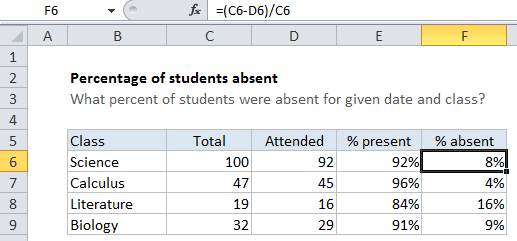# How to calculate percent of students absent in Excel

To calculate percent of students attending a class, given a total class size and the number of students attending, you can use a simple formula that divides students absent (calculated by subtracting attending from total) by the total. The result must be formatted using the percentage number format.

## Formula

`=(total-attended)/total`Note: when working manually, percentage results are calculated by dividing one number by another (for example, a part by a whole) to get a decimal value, then multiplying by 100 to express as a percentage. However, because Excel can display decimal or fractional values automatically as a percentage by applying the Percentage number format, there is no need to multiply by 100.

Also See:   How to get amount with percentage in Excel

## Explanation

In the example shown, we have a total class size in column C, total attended in column D, the calculated percent attending in Column E, and the calculated percent absent in column F.

In cell F6, the formula is:

`=(C6-D6)/C6`

### How this formula works

The formula in F5 first calculates the number of students absent using (C6-D6), then divides the result by the total students. So the formula reduces like this:

```=(C6-D6)/C6
=(100-92)/100
=8/100
=.08```

When formatted using the Percentage number format .08 = 8%.